Written by Paul Bourke
January 2017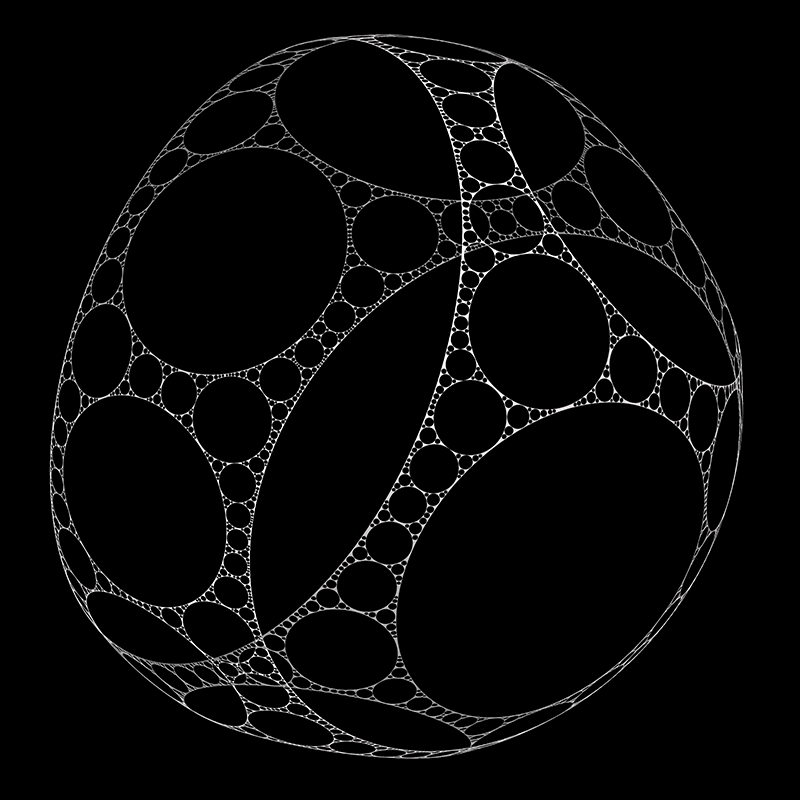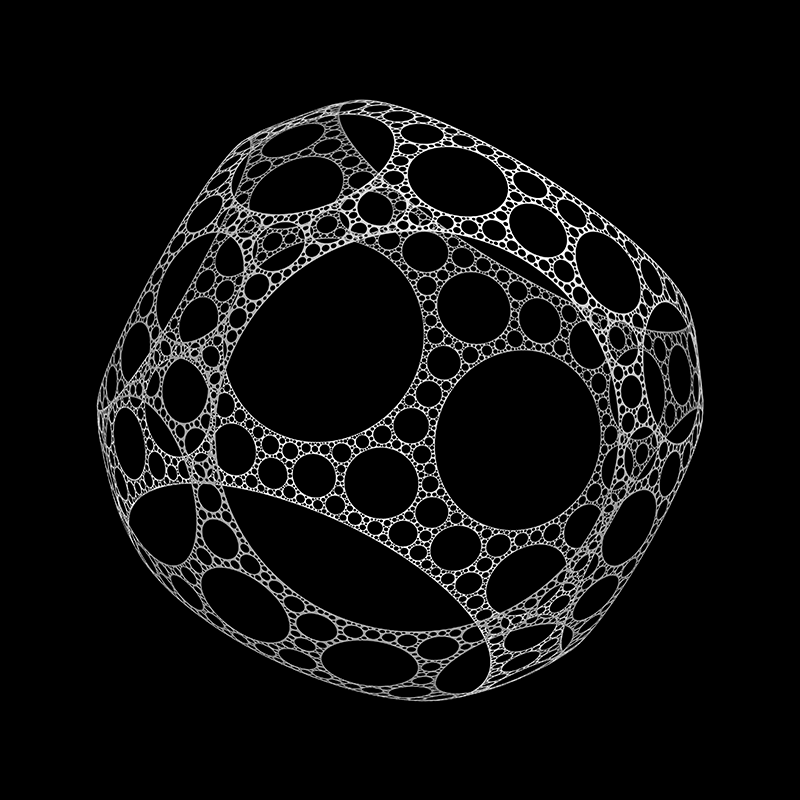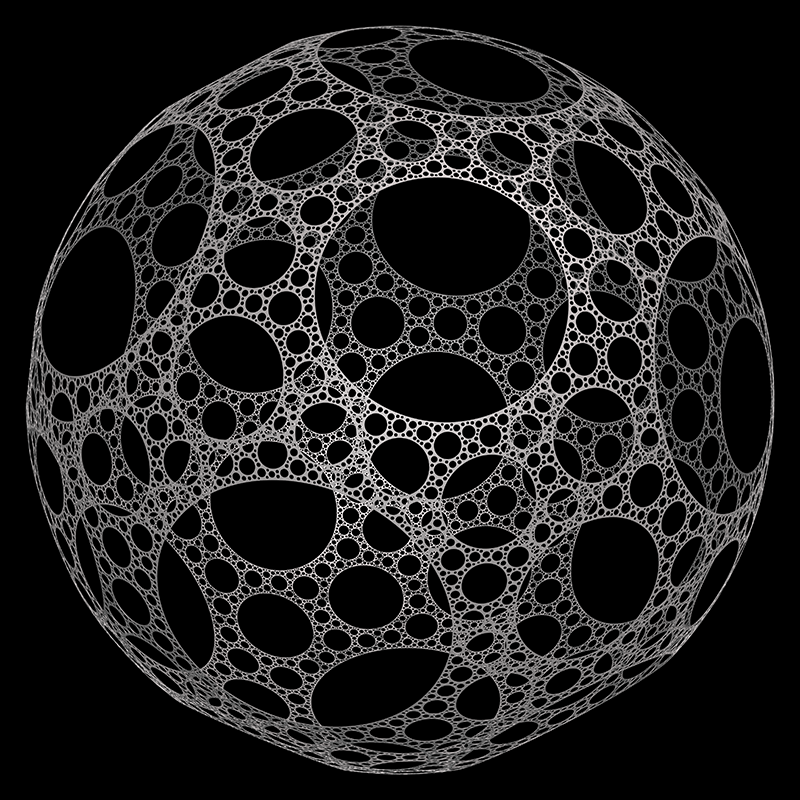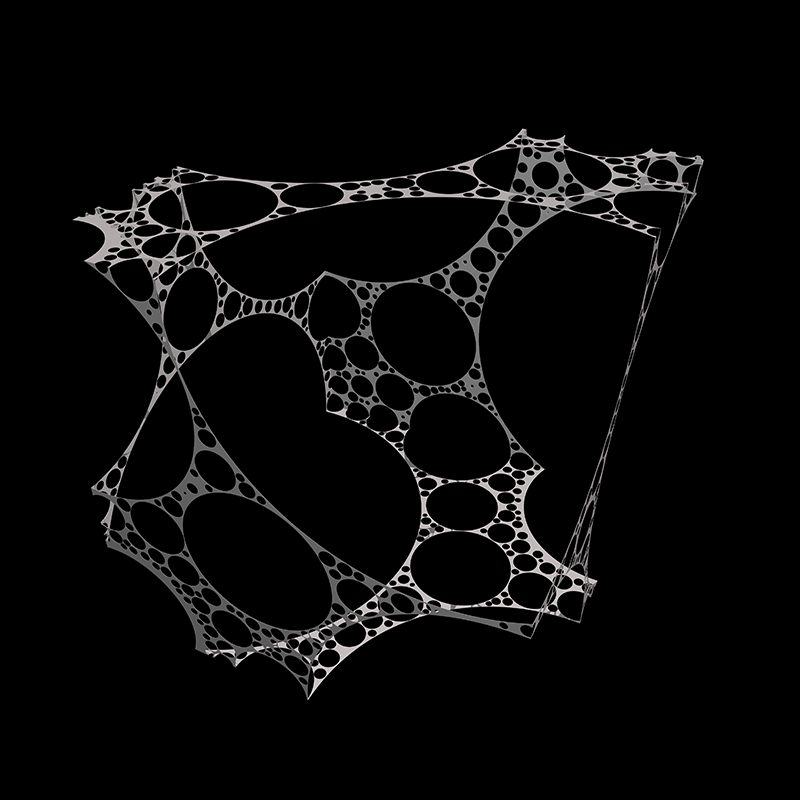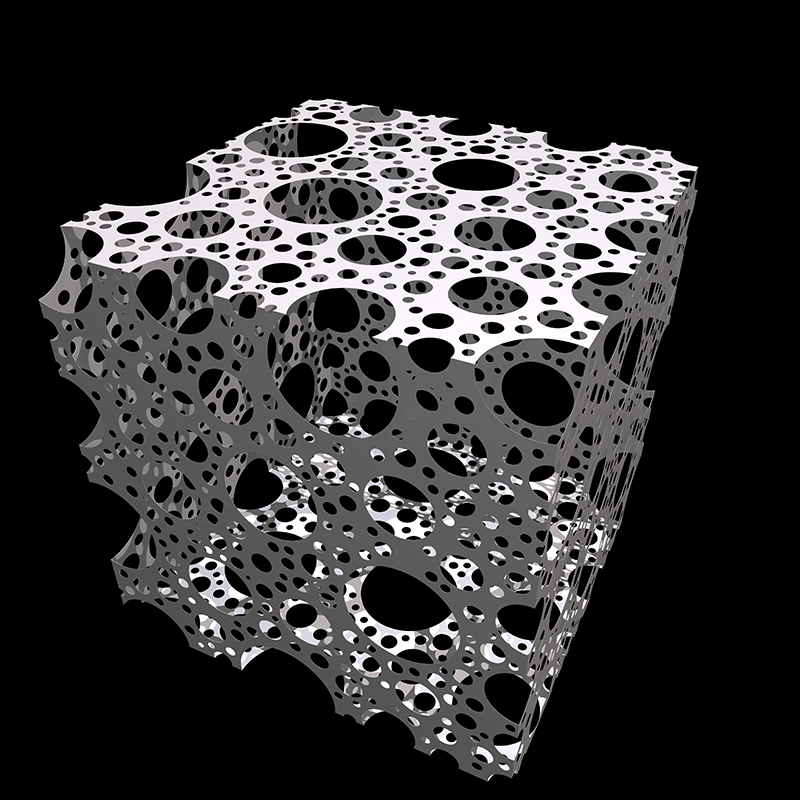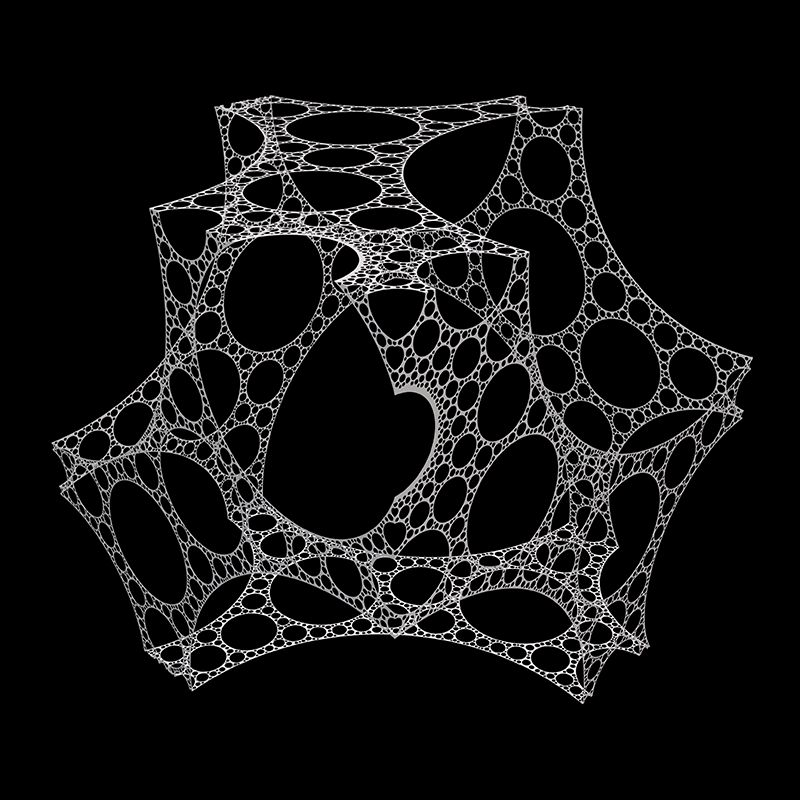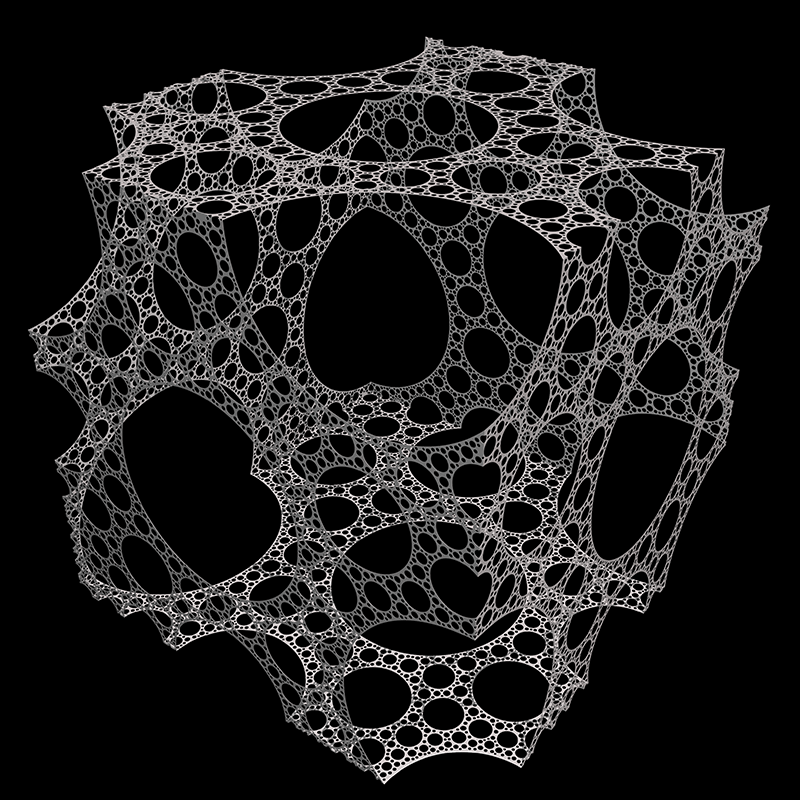Procedure, start with a shape, eg: sphere. Start with an initial subtracting sphere and subtract that from a random position on the starting shape. Search for another random position that does not intersect any of the subtracting shapes so far. If one is found then subtract and repeat. If a spot cannot be found reduce the radius of the subtracting sphere and repeat. This is similar to the work of John Shier except he found the formula which gives the reduction factor to ensure space filling.